# Keesom potential

The Keesom potential is a Boltzmann average over the dipolar section of the Stockmayer potential, resulting in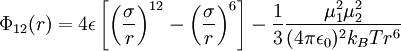$\Phi_{12}(r) = 4 \epsilon \left[ \left(\frac{\sigma}{r} \right)^{12}- \left( \frac{\sigma}{r}\right)^6 \right] - \frac{1}{3}\frac{\mu^2_1 \mu^2_2}{(4\pi\epsilon_0)^2 k_BT r^6}$

where:

•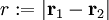$r := |\mathbf{r}_1 - \mathbf{r}_2|$
•$\Phi_{12}(r)$ is the intermolecular pair potential between two particles at a distance r;
•$\sigma$ is the diameter (length), i.e. the value of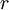$r$ at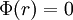$\Phi(r)=0$ ;
•$\epsilon$ : well depth (energy)
•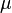$\mu$ is the dipole moment
•$T$ is the temperature
•$k_B$ is the Boltzmann constant
•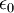$\epsilon_0$ is the permitiviy of free space.

For dipoles dissolved in a dielectric medium, this equation may be generalized by including the dielectric constant of the medium within the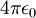$4\pi\epsilon_0$ term.